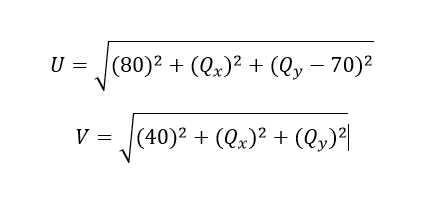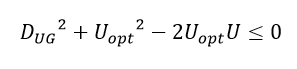# Initialize Variables with Certain Value

How can I initialize variables at the start of CVX?
as I was running the code, and the successive access method is used, I found out that the first variable started near zero while the second variable started near 2e^300. Is there any way for me to initialize the 2 variables with certain values? like Qx =-500 and Qy= 20 and start the iteration from there.
cvx_begin
variable Qx
variable Qy
for i=2:3
Qx_old=Qx_new;
Qy_old=Qy_new;
U=sqrt(power(2,Qx)+power(2,norm([80 Qy-70])));
V=sqrt(power(2,Qx)+power(2,norm([40 Qy])));
rate=rate+((Bo(i)/(Ao(i)log(2)))U+(Co(i)/(Ao(i)log(2)))V);
Qx_new=Qx;
Qy_new=Qy;
subject to
Qx<=500;
Qx>=-500;
power(2,norm([D_UG(i-1) norm([Uopt sqrt(2
U
508)])]))<=0;
power(2,norm([D_UR(i-1) norm([Vopt sqrt(2
V
502)])]))<=0;
power(2,norm([Qx_new-Qx_old Qy_new-Qy_old]))<=625;

end

Thank you

CVX variables can’t be initialized by the user. How do you even know what values the solver started your variables at?

Your program is very crazy looking, and seems unlikely to be what you really want, although I have no idea what that is.

What is the successive access method you refer to? Apparently, it is not CVX’s Successive Approximation method. Not is it obviously Successive Convex Approximation (SCA), unless there is part of your program you are not showing.

Your program has no minimize or maximize statement. Therefore, it is a feasibility problem. I.e., any feasible solution is optimal.

You appear to be doing something crazy with Qx_old, Qx_new, Qy_old, Qy_new. if you are trying to do SCA, the fixing of variables and convexifying around the fixed values, needs to be outside cvx_begin … cvx_end, not inside it. if you use SCA, you can choose the starting value for the “fixed” variable(s), because that is input data to the CVX optimization problem, and those are not (optimization) variables as considered by CVX.

Yes I did not write her the whole code. the point is that I want to write down the functions in the attached image.However, I tried many ways to write these equations till I reached to these formulas

``````U=sqrt(power(2,Qx)+power(2,norm([80 Qy-70]))) ,V=sqrt(power(2,Qx)+power(2,norm([40 Qy])))
``````

given that my variables are Qx and Qy.

The thing is I got a non-logical solution. So my question is there a perfect way to write these formulas?

Thank you for your help, I really appreciate it.

``````U = norm([80;Qx;Qy-70]);
V = norm([40;Qx;Qy]);``````

thank youuu
so how can I write this constraint like the attached photogiven that, U is convex and D_UG and U_opt are constant integers.

If Uopt is positive, that constraint is not convex. If Uopt is negative, you can enter the constraint “as is”.